# 矩阵分解 (特征值/奇异值分解+SVD+解齐次/非齐次线性方程组)

30 篇文章 2 订阅

，#1. 用途#

## 1.1 应用领域

• 最优化问题：最小二乘问题 (求取最小二乘解的方法一般使用SVD)
• 统计分析：信号与图像处理
• 求解线性方程组 Ax=0Ax=b $Ax = 0 或 Ax =b$
• 奇异值分解：可以降维，同时可以降低数据存储需求

## 1.2 矩阵是什么

• 矩阵是什么取决于应用场景
• 矩阵可以是：
• 只是一堆数：如果不对这堆数建立一些运算规则
• 矩阵是一列列向量：如果每一列向量列举了对同一个客观事物的多方面的观察值
• 矩阵是一个图像：它的每个元素代表对应位置的像素值
• 矩阵是一个线性变换：它可以将一些向量变换为另一些向量

## 1.3 矩阵与线性变换

• 矩阵的本质：矩阵的本质就是线性变换

• 基-坐标系一个基定义了一个坐标系

• 矩阵-线性变换：在线性空间中，当选定一组基（相当于确定坐标系）之后，不仅可以用一个向量来描述空间中的任何一个对象，而且可以用矩阵来描述此空间中的任何一个运行（变换），即任何一个线性变换， 都可以用一个确定的矩阵来加以描述

• 向量：向量描述对象（在选定基之后）

• 矩阵：矩阵描述对象的运动（在选定基之后）
• 运动：使某个对象发生要求的运动，就是用描述此运动的矩阵乘以运动对象的向量（运动 * 对象 = 矩阵 * 向量）
• 特征值-变换：同一个线性变换在同的坐标系（基）下的矩阵不同，但其本质相同， 所以特征值相同
• 矩阵可进行哪些线性变换？
• 旋转
• 缩放
• 投影
• 矩阵包含这么多功能，当我们看着一个数据表，对它的功能一无所知，很是迷茫，为了达到我们人类的目的，大神们把它进行分解，从而达到我们人类理解、使用的目标。
• 特征向量：都是正交的，即相互垂直
• 特征值分解和奇异值分解：都是给一个矩阵(线性变换)找一组特殊的基
• 特征值分解：找到了特征向量这一组基，在这组基下该线性变换只有缩放效果
• 奇异值分解(SVD)：则是找到两组基，从一组基到另一组的线性变换的旋转、缩放、投影三种功能独立地展示出来了

# 2. 特征值分解-方阵

• 只有方阵才能进行特征值分解

• 奇异值和特征值的重要意义相似，都是为了提取出矩阵的主要特征

• 特征值的本质 Ax=λx $Ax=\lambda x$

• 特征值分解：把方阵分解为缩放矩阵+特征向量矩阵，没有旋转或旋转角度为0

• 特征值-变化的主次：如果我们想要描述好一个变换，那我们就描述好这个变换主要的变化方向就好了。反过头来看看之前特征值分解的式子，分解得到的 $\land$矩阵是一个对角阵，里面的特征值是由大到小排列的，这些特征值所对应的特征向量就是描述这个矩阵变化方向（从主要的变化到次要的变化排列）

• 高维线性变换：当矩阵是高维的情况下，那么这个矩阵就是高维空间下的一个线性变换，这个线性变化可能没法通过图片来表示，但是可以想象，这个变换也同样有很多的变换方向，我们通过特征值分解得到的前N个特征向量，那么就对应了这个矩阵最主要的N个变化方向。我们利用这前N个变化方向，就可以近似这个矩阵（变换）。也就是之前说的：提取这个矩阵最重要的特征

• 特征值分解总结：特征值分解可以得到：

• 特征值：特征值表示的是这个特征到底有多重要
• 特征向量：而特征向量表示这个特征是什么，可以将每一个特征向量理解为一个线性的子空间，我们可以利用这些线性的子空间干很多的事情。
• 特征值分解的局限：特征值分解也有很多的局限，比如说变换的矩阵必须是方阵。

## 2.1 方阵的分解

• ARn×n $A \in R^{n \times n}$，则A可表示为：

A=XX1 $A = X \land X^{-1}$

• X的列：为A的特征向量

• $\land$为对角矩阵：对角线上的值为A的特征值，按从大到小的顺序排列

## 2.2 实对称矩阵的分解

• SRn×n $S \in R^{n \times n}$，且是对称矩阵，则S可表示为：

S=UUT $S = U \land U^{T}$

• U的列：为S的单位正交特征向量，即U是正交矩阵（列/行向量正交性、归一化，且 U1=UT $U^{-1} = U^{T}$

• $\land$为对角矩阵：对角线上的值为S的特征值，按从大到小的顺序排列
• U就是矩阵A所定义的坐标系：U的n个列向量组成A的一个完备的标准正交特征向量系

# 3. 奇异值分解(SVD) - 非方阵

• 只有非方阵才能进行奇异值分解

• SVD分解：把矩阵分解为缩放矩阵+旋转矩阵+特征向量矩阵

• A的非0奇异值的个数等于它的秩 r $r$

## 3.1 SVD定义

• ARm×n$A \in R^{m \times n}$，且 rank(A) $rank(A)$ = r $r$ (r$r$ > 0)，则矩阵A的奇异值分解(SVD)可表示为：

A=UΣVT $A=U \Sigma V^T$

A=U[Σ000]VT=σ1u1vT1+σ2u2vT2+σrurvTr

• U $U$V$V$都为正交矩阵

• 几何含义

• 表示找到了 U $U$V$V$这样两组基：A矩阵的作用是将一个向量从 V $V$这组正交基向量的空间旋转U$U$这组正交基向量的空间，并对每个方向进行了一定的缩放(由 Σ $\Sigma$决定），缩放因子就是各个奇异值。如果 V $V$的维度比U$U$ 大，则表示还进行了投影
• 奇异值分解：将一个矩阵原本混合在一起的三种作用效果，分解出来了。
• SVD分解如下图所示：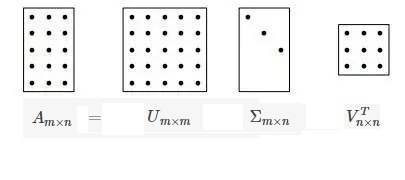• URm×m $U \in R^{m \times m}$（左奇异向量）： U $U$的列为AAT$AA^T$的正交特征向量

• VRn×n $V \in R^{n \times n}$（右奇异向量）： V $V$的列为ATA$A^TA$的正交特征向量

• AAT $AA^T$ ATA $A^TA$：是实对称正定矩阵，且其特征值为非负实数

• rank( AAT $AA^T$) = rank( ATA $A^TA$) = rank(A)

• AAT $AA^T$ ATA $A^TA$的特征值相同：为 λ1,λ2,...,λr $\lambda_1, \lambda_2, ..., \lambda_r$，且 λiλi+1λi0 $\lambda_i \ge \lambda_{i+1}， \lambda_i \ge 0$

• ΣRm×n $\Sigma \in R^{m \times n}$ σi=Σii=λi $\sigma_i = \Sigma_{ii} = \sqrt{\lambda_i}$，其它元素的值为0

• Σ $\Sigma$ = diag(σ1,σ2,...,σr) $diag(\sigma_1, \sigma_2, ..., \sigma_r)$

• σi(i=1,2,...,r)σ1...σr>0 $\sigma_i (i=1,2,...,r)， \sigma_1 \ge ... \ge \sigma_r > 0$：为矩阵A的全部奇异值

• 奇异值σ跟特征值类似，在矩阵Σ中也是从大到小排列，而且σ的减少特别的快，在很多情况下，前10%甚至1%的奇异值的和就占了全部的奇异值之和的99%以上了。也就是说，我们也可以用前 k $k$个大的奇异值来近似描述矩阵，这里定义一下部分奇异值分解：

Am×nUm×kΣk×kVTk×n

• 右边的三个矩阵相乘的结果将会是一个接近于A的矩阵，在这儿，k越接近于n，则相乘的结果越接近于A。而这三个矩阵的面积之和（在存储观点来说，矩阵面积越小，存储量就越小）要远远小于原始的矩阵A，我们如果想要压缩空间来表示原矩阵A，我们存下这里的三个矩阵：U、Σ、V就好了。

## 3.2 SVD特征

• 奇异值的比例不变性
αA $\alpha A$的奇异值是A的奇异值的 |α| $|\alpha|$

• 奇异值的旋转不变性
P $P$是正交矩阵且detA=1$detA=1$(即 P $P$为旋转矩阵)，PA$PA$的奇异值与 A $A$的奇异值相同

• 奇异值的比例和旋转不变性：在数字图像的旋转、镜像、平移、放大、缩小等几何变换方面有很好的应用

• 容易得到矩阵A$A$的秩为 k $k$(kr$k \le r$)的一个最佳逼近矩阵

• 这个特性可以应用于信号的分解和重构， 提取有用信息，消除信号噪声
A=UΣVT=σ1u1vT1+σ2u2vT2+σrurvTr
• 权系数大的哪些项对矩阵 A $A$的贡献大，因此当舍去权系数小的一些项后，仍然能较好地接近矩阵A$A$，这一点在数字图像处理方面非常有用。

• 矩阵 A $A$的秩k$k$逼近定义为：

A=σ1u1vT1+σ2u2vT2+σkukvTk(1kr)

• r $r$逼近就精确等于A$A$，而秩 1 $1$逼近的误差最大

# 4. 齐次/非齐次线性方程组

• 矩阵Am×n$A_{m \times n}$
• 方程组数： m $m$
• 未知数的数量：n$n$
• 齐次线性方程组 Ax=0 $Ax = 0$
• 如果 m<n $m (行数小于列数，即未知数的数量大于所给方程组数），则齐次线性方程组有非零解。
• 齐次线性方程组的两个解的和仍是齐次线性方程组的一组解（加法封闭）
• 齐次线性方程组的解的k倍仍然是齐次线性方程组的解（乘法封闭）
• 齐次线性方程组的系数矩阵秩 rank(A)=ndetA0 $rank(A)=n （detA \neq 0）$，方程组有唯一零解
• 齐次线性方程组的系数矩阵秩 rank(A)<ndetA=0 $rank(A)，方程组有无数多解
• 齐次线性方程组有非零解的充要条件是其系数行列式( det $det$)为零。等价地，方程组有唯一的零解的充要条件是系数矩阵不为零
• 非齐次线性方程组 Ax=b(b0) $Ax = b \quad (b \neq 0)$
• 非齐次线性方程组 有解的充分必要条件是：系数矩阵的秩等于增广矩阵的秩，即 rank(A)=rank(A|b) $rank(A)=rank(A \;|\; b)$（否则为无解）
• 唯一解的充要条件是 rank(A)=n $rank(A)=n$
• 无穷多解的充要条件是 rank(A)<n $rank(A)
• 解的结构：非齐次线性方程组的通解=齐次线性方程组的通解+非齐次线性方程组的一个特解
η=ζ+η

# 5. SVD解优化问题

## 5.1 SVD解非齐次线性方程组（ Ax=b $Ax = b$）

• 求解非齐次线性方程组 Ax=b $Ax=b$
• Am×n $A_{m \times n}$

• m<n $m: 方程个数小于未知变量个数，无唯一解
• m=n $m=n$：若A可逆（ detA0 $detA \neq 0$ 或 rank(A) = n），有唯一解
• m>n $m>n$：方程个数多于未知变量个数，若 rank(A)=rank(A|b) $rank(A)=rank(A \;|\; b)$，则有解
• 对于 m>n $m>n$， 有如下几种情况
1） r(A)<r(A|b) $r(A)： 方程组无解
2） r(A)=r(A|b)=n $r(A)=r(A | b) =n$：方程组有唯一解（约束较强
3） r(A)=r(A|b)<n $r(A)=r(A | b) ：方程组无穷解（约束不够
4） r(A)>r(A|b) $r(A)>r(A | b)$： 不可能，因为增广矩阵的秩大于等于系数矩阵的秩（在矩阵中加入一列，其秩只可能增大，不可能变小）
• M为正交矩阵, x $x$为列向量：则有||Mx||2=||x||2||Mx||=||x||$||Mx||_2 = ||x||_2 或记为：||Mx|| = ||x||$

• ||x||2=||x||=xTx $||x||_2 = ||x|| = \sqrt{x^Tx}$：即向量 x $x$的长度
• 以下讨论前提为：mn$m \geqslant n$

• 等价于寻找 x $x$使||Axb||2$||Ax -b||_2$最小化 （向量2范数，转化为最优化问题）

1) 对矩阵A进行SVD分解( Dm×n $D_{m \times n}$对角阵，且 rank(A)=n $rank(A) = n$)：

A=UDVT

则优化问题变为：
min(||Axb||2)=min(||UDVTxb||2)=min(||DVTxUTb||2)

2) 令：

y=VTxb=UTb

则优化问题变为：
min(||DVTxUTb||2)=min(||Dyb||2)

3) 求解向量 y $y$

• Dyb=0$D y-b' =0$，即 Dy=b $Dy=b'$，其方程组形式如下图所示：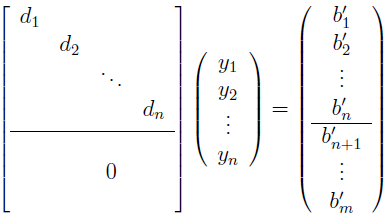• 则有： yi=bi/di(i=0,1,...,rank(A)) $y_i = b_i'/d_i \quad (i=0,1,...,rank(A))$

4) 求解向量 x $x$

x=Vy

### 5.1.1 rank(A) = n的求解步骤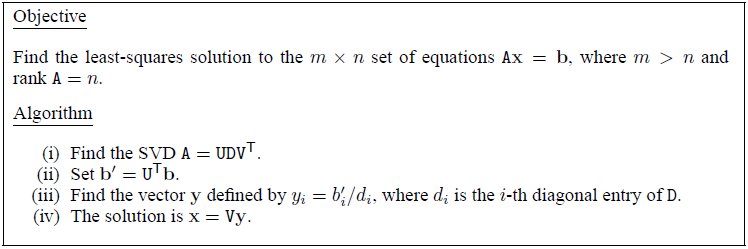### 5.1.2 rank(A) < n的求解步骤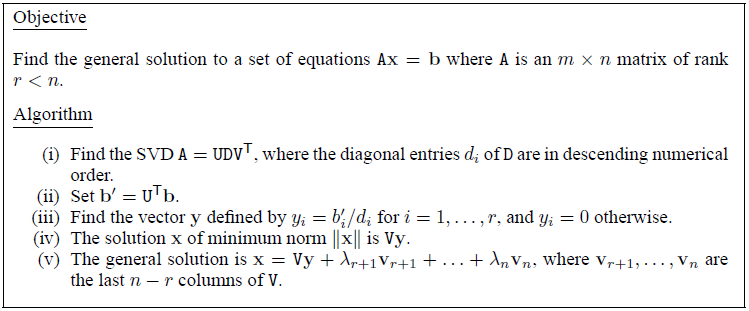• λi $\lambda _i$：是用于参数化的随机值（parametrized by the indeterminate values

## 5.2 SVD解齐次线性方程组（ Ax=0 $Ax = 0$）

• 相似的情况，我们把问题转化为最小化 ||Ax||2 $|| Ax ||_2$的非线性优化问题，我们已经知道了 x=0 $x = 0$是该方程组的一个特解，为了避免 x=0 $x = 0$这种情况（因为在实际的应用中 x=0 $x = 0$往往不是我们想要的），我们增加一个约束，比如 ||x||2=1 $|| x ||_2 = 1$，这样，问题就变为（带约束的优化问题 s.t. : subject to）：
min||Ax||s.t.||x||=1

min||Ax||=min||UDVTx||=min||DVTx||||x||=||VTx||

y=VTxmin||Dy||s.t.:||y||=1V
• 由于D是一个对角矩阵，对角元素按降序排列，因此最优解在 y=(0,0,...,1)T $y = (0, 0,..., 1)^T$时取得，又因为 x=Vy $x = Vy$， 所以最优解就是V的最小奇异值对应的列向量，比如，最小奇异值在第6行6列，那么x 为 V的第6个列向量。
• 求解步骤：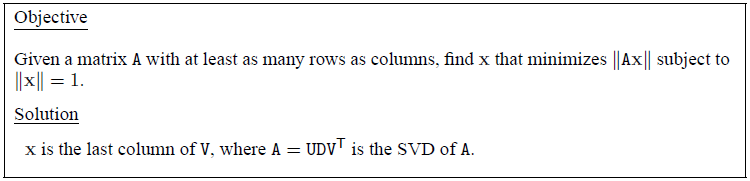09-30点击重新获取扫码支付余额充值